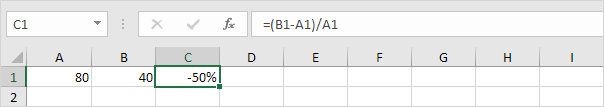# How to Calculating percentages with Formulas in Excel

Feb 01, 2020 • edited Feb 02, 2020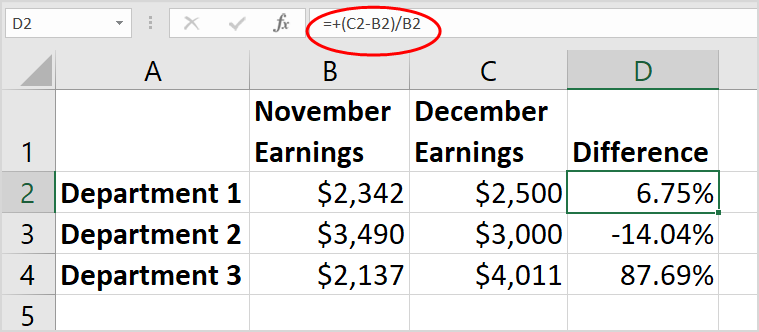How to calculate percentages in Excel. Learn how to show data effectively for reports for example when calculating percentage change (percent increase or decrease) from Actual to Budget or this year to previous year.

Also learn how to calculate the final amount after a percent increase or percent decrease. For example if you have a 10% price increase - what is your final value?

Calculating percentages in Excel is easy. Percentage simply means ‘out of 100’, so 72% is ‘72 out of 100’ and 4% is ‘4 out of 100’, etc.

### Enter a Percentage

To enter a percentage in Excel, execute the following steps.

1. First, enter a decimal number.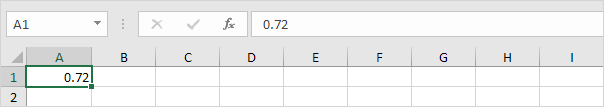2. On the Home tab, in the Number group, click the percentage symbol to apply a Percentage format.Result.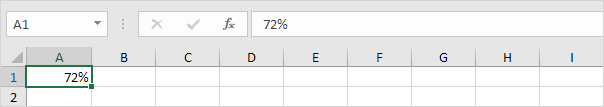Note: to change the percentage in cell A1, simply select cell A1 and type a new percentage (do not type a decimal number).

### Percentage of Total

To calculate the percentage of a total in Excel, execute the following steps.

1. Enter the formula shown below. This formula divides the value in cell A1 by the value in cell B1. Simply use the forward slash (/) as the division operator. Don't forget, always start a formula with an equal sign (=).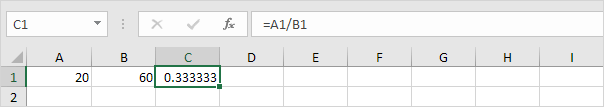2. On the Home tab, in the Number group, click the percentage symbol to apply a Percentage format.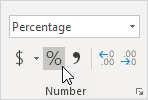Result.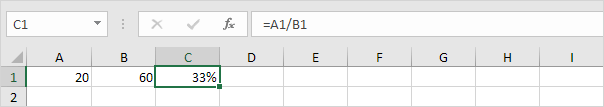3. On the Home tab, in the Number group, click the Increase Decimal button once.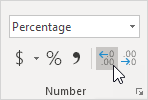Result.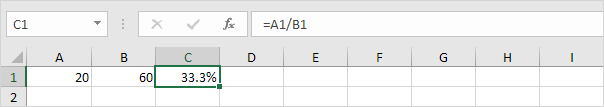Note: Excel always uses the underlying precise value in calculations, regardless of how many decimals you choose to display.

### Increase by Percentage

To increase a number by a percentage in Excel, execute the following steps.

1. Enter a number in cell A1. Enter a decimal number (0.2) in cell B1 and apply a Percentage format.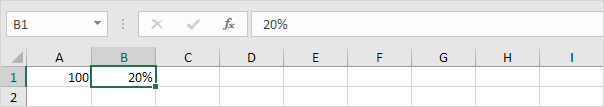2. To increase the number in cell A1 by 20%, multiply the number by 1.2 (1+0.2). The formula below does the trick.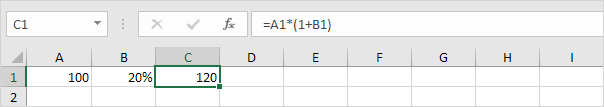Note: Excel uses a default order in which calculations occur. If a part of the formula is in parentheses, that part will be calculated first.

3. To decrease a number by a percentage, simply change the plus sign to a minus sign.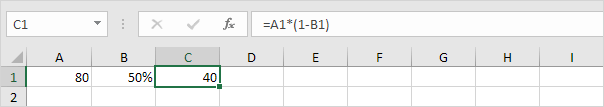### Percentage Change

To calculate the percentage change between two numbers in Excel, execute the following steps.

1. Enter an old number in cell A1 and a new number in cell B1.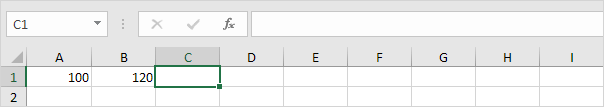2. First, calculate the difference between new and old.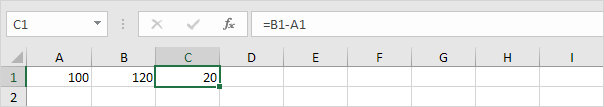3. Next, divide this result by the old number in cell A1.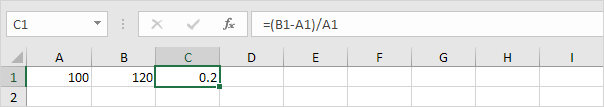Note: Excel uses a default order in which calculations occur. If a part of the formula is in parentheses, that part will be calculated first.

4. On the Home tab, in the Number group, click the percentage symbol to apply a Percentage format.Result.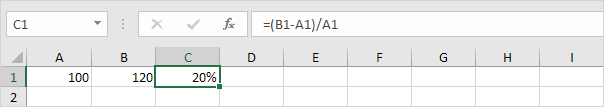5. The (New-Old)/Old formula always works.